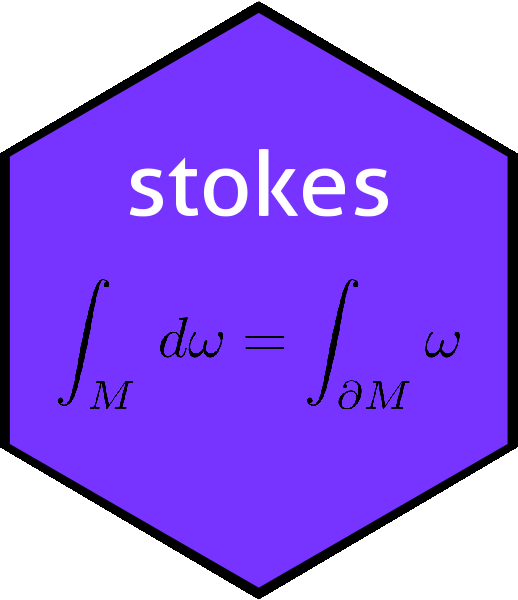# Function vector_cross_product() in the stokes packagevector_cross_product
## function (M)
## {
##     n <- nrow(M)
##     stopifnot(n == ncol(M) + 1)
##     (-1)^n * sapply(seq_len(n), function(i) {
##         (-1)^i * det(M[-i, ])
##     })
## }
## <bytecode: 0x7fc3abfdc040>
## <environment: namespace:stokes>

# The vector cross product

Spivak (p83) considers the standard vector cross product $$\mathbf{u}\times\mathbf{v}=\det\begin{pmatrix} i & j & k \\ u_1&u_2&u_3\\ v_1&v_2&v_3 \end{pmatrix}$$ and places it in a more general and rigorous context. In a memorable passage, he states:

If $$v_1,\ldots,v_{n-1}\in\mathbb{R}^n$$ and $$\phi$$ is defined by

$\phi(w)=\det\left(\begin{array}{c}v_1\\ \vdots\\ v_{n-1}\\w\end{array}\right)$

then $$\phi\in\Lambda^1\left(\mathbb{R}^n\right)$$; therefore there is a unique $$z\in\mathbb{R}^n$$ such that

$\left\langle w,z\right\rangle=\phi(w)= \det\left(\begin{array}{c}v_1\\ \vdots\\ v_{n-1}\\w\end{array}\right).$

This $$z$$ is denoted $$v_1\times\ldots\times v_{n-1}$$ and is called the cross product of $$v_1,\ldots,v_{n-1}$$.

- Michael Spivak, 1969 (Calculus on Manifolds, Perseus books). Pages 83-84

The reason that $$\mathbf{w}$$ is at the bottom rather than the top is that it ensures that the the $$n$$-tuple $$(\mathbf{v}_1,\ldots,\mathbf{v}_{n-1},\mathbf{w})$$ has positive orientation with respect to the standard basis vectors of $$\mathbb{R}^n$$. In $$\mathbb{R}^3$$ we get the standard elementary mnemonic for $$\mathbf{u}=(u_1,u_2,u_3)$$, $$\mathbf{v}=(v_1,v_2,v_3)$$:

$\mathbf{u}\times\mathbf{v}= \mathrm{det} \begin{pmatrix} i&j&k\\ u_1&u_2&u_3\\ v_1&v_2&v_3 \end{pmatrix}$

## R implementation

The R function takes a matrix with $$n$$ rows and $$n-1$$ columns: the transpose of the work above. This is because stokes (and R) convention is to interpret columns of a matrix as vectors. If we wanted to take the cross product of $$\mathbf{u}=(5,-2,1)$$ with $$\mathbf{v}=(1,2,0)$$:

(M <- cbind(c(5,-2,1),c(1,2,0)))
##      [,1] [,2]
## [1,]    5    1
## [2,]   -2    2
## [3,]    1    0
vector_cross_product(M)
##  -2  1 12

But of course we can work with higher dimensional spaces:

vector_cross_product(matrix(rnorm(30),6,5))
##  -7.892174  0.927769  1.070595 -5.334296  1.771056 -4.517277

# Verification

We can demonstrate that the function has the correct orientation. We need to ensure that the vectors $$\mathbf{v}_1,\ldots,\mathbf{v}_n,\mathbf{v}_1\times\cdots\times\mathbf{v}_n$$ constitute a right-handed basis:

det(cbind(M,vector_cross_product(M)))>0
##  TRUE

So it is right-handed in this case. Here is a more severe test:

f <- function(n){
M <- matrix(rnorm(n^2+n),n+1,n)
det(cbind(M,vector_cross_product(M)))>0
}

all(sapply(sample(3:10,100,replace=TRUE),f))
##  TRUE

### Vector products and Hodge

The cross product has a coordinate-free definition as the Hodge conjugate of the wedge product of its arguments. This is not used in function vector_cross_product() because it is computationally inefficient and (I think) prone to numerical roundoff errors. We may verify that the definitions agree, using a six-dimensional test case:

set.seed(2)
M <- matrix(rnorm(30),6,5)
(ans1 <- vector_cross_product(M))
##    4.431826  -1.966102  -3.344998  -6.853352 -11.879641   7.170485

We can see that vector_cross_product() returns an R vector. To verify that this is correct, we compare the output with the value calculated directly with the wedge product:

hodge(as.1form(M[,1]) ^ as.1form(M[,2]) ^ as.1form(M[,3]) ^ as.1form(M[,4]) ^ as.1form(M[,5]))
## An alternating linear map from V^1 to R with V=R^6:
##               val
##  6  =    7.170485
##  1  =    4.431826
##  3  =   -3.344998
##  4  =   -6.853352
##  2  =   -1.966103
##  5  =  -11.879641

Actually it is possible to produce the same answer using slightly slicker idiom:

(ans2 <- hodge(Reduce(^,lapply(1:5,function(i){as.1form(M[,i])}))))
## An alternating linear map from V^1 to R with V=R^6:
##               val
##  2  =   -1.966103
##  6  =    7.170485
##  3  =   -3.344998
##  5  =  -11.879641
##  4  =   -6.853352
##  1  =    4.431826

[again note the different order in the output]. Above, we see that the output of vector_cross_product() [ans1] is an ordinary R vector, but the direct result [ans2] is a 1-form. In order to compare these, we first need to coerce ans1 to a 1-form and then subtract:

(diff <- as.1form(ans1) - ans2)
## An alternating linear map from V^1 to R with V=R^6:
##        val
##  1  =    0
##  2  =    0
##  3  =    0
##  4  =    0
##  5  =    0
##  6  =    0
coeffs(diff)
## A disord object with hash 8e9298ff6d77253137fe25d06acabd9c369e4321 and elements
##   3.552714e-15  8.881784e-16 -1.776357e-15  8.881784e-16  1.776357e-15
##  -6.217249e-15
## (in some order)

Above we see that ans1 and ans2 match to within numerical precision.

## Vector cross products in 3 dimensions

### Use of the contract() function

Taking Spivak’s definition at face value, we could define the vector cross product $$\mathbf{u}\times\mathbf{v}$$ of three-vectors $$\mathbf{u}$$ and $$\mathbf{v}$$ as a map from the tangent space to the reals, with $$\left(\mathbf{u}\times\mathbf{v}\right)(\mathbf{w})= \left(\mathbf{u}\times\mathbf{v}\right)\cdot\mathbf{w} =\left(I_\mathbf{u}\right)_\mathbf{v}(\mathbf{w})$$, where $$I$$ is the 3-volume element and subscripts refer to contraction. This is easy to implement in the package. Suppose we wish to take the vector cross product of $$\mathbf{u}=\left(1,4,2\right)^T$$ and $$\mathbf{v}=\left(2,1,5\right)^T$$:

u <- c(1,4,2)
v <- c(2,1,5)
(vcp <- contract(volume(3),cbind(u,v)))
## An alternating linear map from V^1 to R with V=R^3:
##        val
##  3  =   -7
##  2  =   -1
##  1  =   18
dovs(vcp)
##  3

Object vcp is the vector cross product of $$\mathbf{u}$$ and $$\mathbf{v}$$, but written a as a one-form. We can see the mnemonic in operation by coercing vcp to a function:

ucv  <- as.function(vcp)

and then evaluating this on the three basis vectors of $$\mathbb{R}^3$$:

c(i=ucv(ex), j=ucv(ey), k=ucv(ez))
##  i  j  k
## 18 -1 -7

and we see agreement with the mnemonic $$\det\begin{pmatrix}i&j&k\\1&4&2\\2&1&5\end{pmatrix}$$.

It is possible to recreate the vector cross product more directly in the package, but we need to coerce the R vectors c(1,4,2) and c(2,1,5) to alternating $$k$$-forms first, using as.1form(). Taking a wedge product gives us a two-form, and to revert to a one form we need to take the hodge dual:

hodge(as.1form(c(1,4,2)) ^ as.1form(c(2,1,5)))
## An alternating linear map from V^1 to R with V=R^3:
##        val
##  3  =   -7
##  2  =   -1
##  1  =   18

Above, note the order of the lines, being implementation-specific as per disordR discipline, may appear in a different order but the form should agree with the previous results.

## Reference

• M. Spivak 1971. Calculus on manifolds, Addison-Wesley.